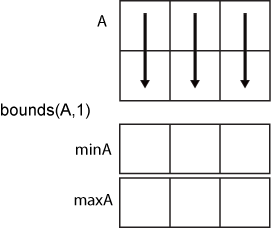# bounds

Minimum and maximum values of an array

## Syntax

``````[minA,maxA] = bounds(A)``````
``[minA,maxA] = bounds(A,'all')``
``````[minA,maxA] = bounds(A,dim)``````
``[minA,maxA] = bounds(A,vecdim)``
``````[minA,maxA] = bounds(___,nanflag)``````

## Description

example

``````[minA,maxA] = bounds(A)``` returns the minimum value `minA` and maximum value `maxA` in an array. `minA` is equivalent to `min(A)` and `maxA` is equivalent to `max(A)`.```

example

````[minA,maxA] = bounds(A,'all')` computes the minimum and maximum values over all elements of `A`. This syntax is valid for MATLAB® versions R2018b and later.```

example

``````[minA,maxA] = bounds(A,dim)``` operates along the dimension `dim` of `A`. For example, if `A` is a matrix, then `bounds(A,2)` returns column vectors `minA` and `maxA` containing the minimum and maximum values in each row.```

example

````[minA,maxA] = bounds(A,vecdim)` computes the minimum and maximum values based on the dimensions specified in the vector `vecdim`. For example, if `A` is a matrix, then `bounds(A,[1 2])` returns the minimum and maximum values over all elements in `A`, since every element of a matrix is contained in the array slice defined by dimensions 1 and 2.```

example

``````[minA,maxA] = bounds(___,nanflag)``` specifies whether to include or omit `NaN` values when determining the minimum and maximum values. `bounds(A,'omitnan')` ignores `NaN` values. If any element of `A` is `NaN`, then `bounds(A,'includenan')` returns `NaN` for both `minA` and `maxA`. The default behavior is `'omitnan'`.```

## Examples

collapse all

Simultaneously compute the minimum and maximum values of a vector.

```A = [2 4 -1 10 6 3 0 -16]; [minA,maxA] = bounds(A)```
```minA = -16 ```
```maxA = 10 ```

Compute the minimum and maximum values in each row of a matrix.

`A = magic(4)`
```A = 4×4 16 2 3 13 5 11 10 8 9 7 6 12 4 14 15 1 ```
`[minA,maxA] = bounds(A,2)`
```minA = 4×1 2 5 6 1 ```
```maxA = 4×1 16 11 12 15 ```

Create a 3-D array and compute the minimum and maximum values in each page of data (rows and columns).

```A(:,:,1) = [2 4; -2 1]; A(:,:,2) = [9 13; -5 7]; A(:,:,3) = [4 4; 8 -3]; [minA1,maxA1] = bounds(A,[1 2]); minA1```
```minA1 = minA1(:,:,1) = -2 minA1(:,:,2) = -5 minA1(:,:,3) = -3 ```
`maxA1`
```maxA1 = maxA1(:,:,1) = 4 maxA1(:,:,2) = 13 maxA1(:,:,3) = 8 ```

Starting in R2018b, to compute the bounds over all dimensions of an array, you can either specify each dimension in the vector dimension argument, or use the `'all'` option.

`[minA2,maxA2] = bounds(A,[1 2 3])`
```minA2 = -5 ```
```maxA2 = 13 ```
`[minAall,maxAall] = bounds(A,'all')`
```minAall = -5 ```
```maxAall = 13 ```

Include and ignore `NaN` elements of a vector when computing its minimum and maximum values.

Ignore `NaN` values when computing the minimum and maximum values of a vector, which is the default.

```A = [2 NaN 6 -5 0 NaN 10]; [minA1,maxA1] = bounds(A)```
```minA1 = -5 ```
```maxA1 = 10 ```

Use the `'includenan'` option to include `NaN` values, which causes `bounds` to return `NaN` for both the minimum and maximum values of `A`.

`[minA2,maxA2] = bounds(A,'includenan')`
```minA2 = NaN ```
```maxA2 = NaN ```

## Input Arguments

collapse all

Input array, specified as a vector, matrix, or multidimensional array.

Data Types: `single` | `double` | `int8` | `int16` | `int32` | `int64` | `uint8` | `uint16` | `uint32` | `uint64` | `logical` | `categorical` | `datetime` | `duration`
Complex Number Support: Yes

Dimension to operate along, specified as a positive integer scalar. If you do not specify the dimension, then the default is the first array dimension of size greater than 1.

Consider an `m`-by-`n` input matrix, `A`:

• `bounds(A,1)` computes the minimum and maximum values in each column of `A` and returns two `1`-by-`n` row vectors.• `bounds(A,2)` computes the minimum and maximum values in each row of `A` and returns two `m`-by-`1` column vectors.Vector of dimensions, specified as a vector of positive integers. Each element represents a dimension of the input array. The lengths of the output in the specified operating dimensions are 1, while the others remain the same.

Consider a 2-by-3-by-3 input array, `A`. Then `[minA,maxA] = bounds(A,[1 2])` returns a 1-by-1-by-3 array for both `minA` and `maxA`. The elements of `minA` and `maxA` are the minimum and maximum values in the corresponding page of `A`, respectively.`NaN` condition, specified as one of these values:

• `'omitnan'` — Ignore all `NaN` values in the input. If the input contains only `NaN` values, then `bounds` returns `NaN` for both `minA` and `maxA`.

• `'includenan'` — Include `NaN` values. If any element of the input is `NaN`, then `bounds` returns `NaN` for both `minA` and `maxA`.

## Output Arguments

collapse all

Minimum value, specified as a vector, matrix, or multidimensional array.

Maximum value, specified as a vector, matrix, or multidimensional array.

## Version History

Introduced in R2017a